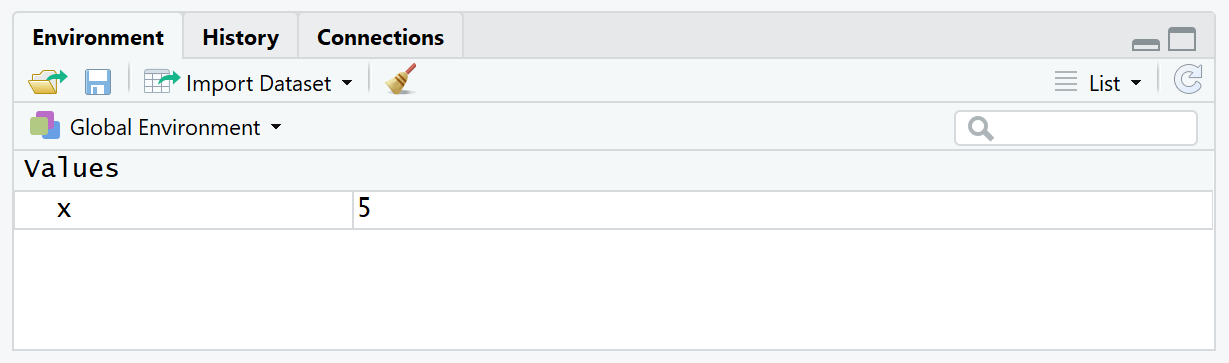# Clear Data Object from Workspace in R (3 Examples) | rm & remove Functions

In this R tutorial you’ll learn how to clear specific data objects from the R workspace. The tutorials relies mainly on the rm() and remove() functions.

The tutorial will consist of these content blocks:

Let’s take a look at some R codes in action!

## Example 1: Remove One Specific Data Object

Let’s assume that we have stored the following data object x in our R workspace:

`x <- 5 # Create data object`Figure 1: R Workspace Contains Data Object x.

Then we can remove this specific data object with the rm function as shown below:

`rm(x) # Apply rm function`Figure 2: Data Object x was Removed from R Workspace.

The advantage of this method is that we can delete specific data objects from our workspace without removing the entire workspace.

## Example 2: Remove Multiple Data Objects

We can also remove several data objects at once with the rm function in R. Consider the following three data objects:

```x <- 5 # Multiple data objects y <- 3 z <- "hello"```

Now, we can simply specify all of these objects within the rm function in order to clear them from the R environment:

`rm(x, y, z) # Remove multiple objects`

## Example 3: rm() vs. remove() Functions

Actually, there are two different functions that can be used for clearing specific data objects from the R workspace: rm() and remove().

However, these two functions are exactly the same. You can use the function you prefer.

For illustration, I’m showing Example 1 based on the remove function:

```x <- 5 # Create data object remove(x) # Apply remove function```

The previous R code also clears the data object x from the R workspace.

## Video, Further Resources & Summary

Would you like to learn more about clearing data objects in R? Then you could have a look at the following video of my YouTube channel. I show the R programming codes of this article in the video.

Furthermore, I can recommend to have a look at the related articles of https://www.statisticsglobe.com/: